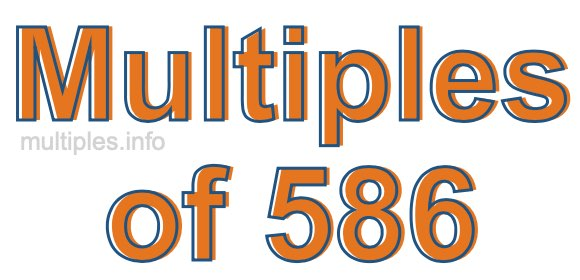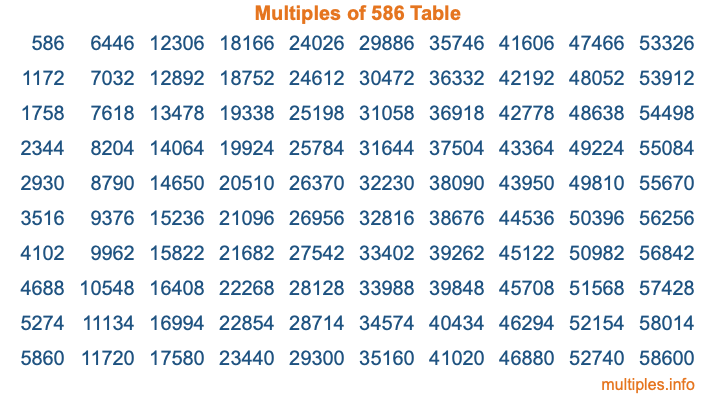Multiples of 586Welcome to the Multiples of 586 page. Here we will first teach you everything you will ever need to know about the multiples of 586, and then give you a study guide summary of everything we taught you to make sure you remember it all. Use this page to look up facts and learn information about the multiples of 586. This page will make you a multiples of five hundred eighty-six expert!

Definition of Multiples of 586
Multiples of 586 are all the numbers that when divided by 586 equal an integer. Each of the multiples of 586 are called a multiple. A multiple of 586 is created by multiplying 586 by an integer.

Therefore, to create a list of multiples of 586, you start with 1 multiplied by 586, then 2 multiplied by 586, then 3 multiplied by 586, and so on for as long as you want. Thus, the list of the first five multiples of 586 is 586, 1172, 1758, 2344, and 2930. To see a larger list of multiples of 586, see the printable image of Multiples of 586 further down on this page. We also have a category where you can choose any nth multiple of 586.

Multiples of 586 Checker
The Multiples of 586 Checker below checks to see if any number of your choice is a multiple of 586. In other words, it checks to see if there is any number (integer) that when multiplied by 586 will equal your number. To do that, we divide your number by 586. If the the quotient is an integer, then your number is a multiple of 586.

Is  a multiple of 586?

Least Common Multiple of 586 and ...
A Least Common Multiple (LCM) is the lowest multiple that two or more numbers have in common. This is also called the smallest common multiple or lowest common multiple and is useful to know when you are adding our subtracting fractions. Enter one or more numbers below (586 is already entered) to find the LCM.

Check out our LCM Calculator if you need more details about the Least Common Multiple or if you need the LCM for different numbers for adding and subtraction fractions.

nth Multiple of 586
As we stated above, 586 is the first multiple of 586, 1172 is the second multiple of 586, 1758 is the third multiple of 586, and so on. Enter a number below to find the nth multiple of 586.

th multiple of 586

Multiples of 586 vs Factors of 586
586 is a multiple of 586 and a factor of 586, but that is where the similarities end. All postive multiples of 586 are 586 or greater than 586. All positive factors of 586 are 586 or less than 586.

Below is the beginning list of multiples of 586 and the factors of 586 so you can compare:

Multiples of 586: 586, 1172, 1758, 2344, 2930, etc.

Factors of 586: 1, 2, 293, 586

As you can see, the multiples of 586 are all the numbers that you can divide by 586 to get a whole number. The factors of 586, on the other hand, are all the whole numbers that you can multiply by another whole number to get 586.

It's also interesting to note that if a number (x) is a factor of 586, then 586 will also be a multiple of that number (x).

Multiples of 586 vs Divisors of 586
The divisors of 586 are all the integers that 586 can be divided by evenly. Below is a list of the divisors of 586.

Divisors of 586: 1, 2, 293, 586

The interesting thing to note here is that if you take any multiple of 586 and divide it by a divisor of 586, you will see that the quotient is an integer.

Multiples of 586 Table
Below is an image of the first 100 multiples of 586 in a table. The table is in chronological order, column by column. The first column has the first ten multiples of 586, the second column has the next ten multiples of 586, and so on.The Multiples of 586 Table is also referred to as the 586 Times Table or Times Table of 586. You are welcome to print out our table for your studies.

Negative Multiples of 586
Although not often discussed or needed in math, it is worth mentioning that you can make a list of negative multiples of 586 by multiplying 586 by -1, then by -2, then by -3, and so on, to get the following list of negative multiples of 586:

-586, -1172, -1758, -2344, -2930, etc.

Multiples of 586 Summary
Below is a summary of important Multiples of 586 facts that we have discussed on this page. To retain the knowledge on this page, we recommend that you read through the summary and explain to yourself or a study partner why they hold true.

There are an infinite number of multiples of 586.

A multiple of 586 divided by 586 will equal a whole number.

586 divided by a factor of 586 equals a divisor of 586.

The nth multiple of 586 is n times 586.

The largest factor of 586 is equal to the first positive multiple of 586.

586 is a multiple of every factor of 586.

586 is a multiple of 586.

A multiple of 586 divided by a divisor of 586 equals an integer.

586 divided by a divisor of 586 equals a factor of 586.

Any integer times 586 will equal a multiple of 586.

Multiples of a Number
Here you can get the multiples of another number, all with the same attention to detail as we did for multiples of 586 on this page.

Multiples of
Multiples of 587
Did you find our page about multiples of five hundred eighty-six educational? Do you want more knowledge? Check out the multiples of the next number on our list!